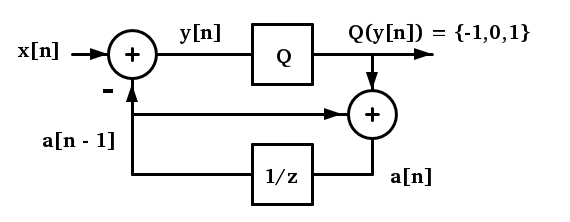## Differential encoder simulationStarted by 5 years ago7 replieslatest reply 5 years ago97 views

Hello,

I am studying the following differential encoder:where the quantizer is defined as $$Q(y) = \text{sgn}(y)$$

and I would like to be sure I understand it correctly, because I have a few doubts.

The analysis I do is the following:

\begin{aligned} \tilde{y}(n) & = Q(y(n))\\ y(n) & = x(n) - a(n-1)\\ a(n) &= \tilde{y}(n) + a(n-1)\\ & = \tilde{x}(n) - a(n-1) + a(n-1)\\ & = \tilde{x}(n)\\ a(n-1) & = \tilde{x}(n-1) \end{aligned}

so if I invent a sequence to be coded, e.g.

$$x(n) = \{0.4,\,0.7,\,1,\,-1.7\}$$

I obtain the following table:

$$\begin{array}{| c | c | c | c | c |}\hline n & 0 & 1 & 2 & 3 \\ \hline x(n) & 0.4 & 0.7 & 1 & -1.7 \\\hline a(n) & 1 & 0 & 1 & 0 \\\hline a(n-1) & 0 & 1 & 0 & 1 \\\hline y(n) & 0.4 & -1.3 & 1 & -2.7 \\\hline \hat{y}(n) & 1 & -1 & 1 & -1 \\\hline \end{array}$$

From the above table I obtain $$a(n) = 0$$ for some values of $$x(n) > 0$$ (e.g. when $$n = 1$$)

If $$a(n) = \tilde{x}(n) = Q(x(n))$$ I suppose it should imply that $$a(n) \neq 0$$ if $$x(n) > 0$$.

So, is there something wrong in my analysis ?

Thank you in advance.

Regards,

Simon

[ - ]
Reply by October 12, 2017Can you elaborate on how you made this substitution?

Where did "x bar" come from?

[ - ]
Reply by October 12, 2017It looks like my copy/paste isn't working. I've got a question about your substitution: "x-bar"(n) - a(n-1) + a(n-1)

Where did "x-bar" come from?

[ - ]
Reply by October 12, 2017Hello @jeff_burch,

You are right. I did not specified it.

I use $$\tilde{x}(n)$$ to represent the quantized value of $$x(n)$$.

So $$\tilde{x}(n) = Q(x(n))$$.

Regards,

Simon

[ - ]
Reply by October 12, 2017Simon,

I think the problem is an incorrect block diagram manipulation. Take your drawing and assume that Q is a linear operator (it isn't, more on that later). You can rework the drawing and place Q to the left and also below the summation point where X[n] enters the drawing. So, your modified drawing would have two Q blocks (both before the summation point).

Let's focus on this second Q block that is in the path between a[n-1] and the summation point. I bet you have assumed that this second Q is superfluous because a[n-1] is already quantized, so you've dropped it from your analysis.

I think you can only legally move Q around like this when it is a linear operator. Because Q is sgn() (i.e. non-linear), I don't think you are allowed to move it around like this.

So, I think you are going astray when you introduced "x-bar" into your equations above. I think you want to make this substitution:

a[n] = sgn( x[n] - a[n-1] ) + a[n-1]

I don't think this can be simplified further. I don't think you can legally distribute the sgn( ) function:

sgn( x[n] - a[n-1])  != sgn( x[n] ) - sgn( a[n-1])

Note, I agree with you that sgn( a[n-1] ) is = a[n-1] but I think the != is still true.

Cheers, Jeff

[ - ]
Reply by October 13, 2017Of course I agree with you. This was another little doubt I had.

Thank you Jeff.

Regards,

Simon

[ - ]
Reply by October 13, 2017Typically when a[n-1] = 1, then the sgn(x[n]-a[n-1]) will be negative for x[n] less than 1.

so for x[n] = 0.7 and a[n-1] = 1, sgn(x[n]-a[n-1]) = -1 even if x[n] is positive.

[ - ]
Reply by October 13, 2017Thank you too @oliviert.

Regards,

Simon